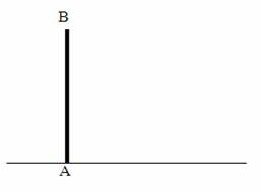## Wednesday, November 30, 2011

### Two Questions (MCQ) on Rotation of Rigid Bodies

“Learn from yesterday, live for today, hope for tomorrow. The important thing is to not stop questioning.”

– Albert Einstein

Today we will discuss a couple of questions involving rigid body rotation. The first question may appear to be familiar to you as it has appeared in various entrance exam question papers. The second one is not so common but you must certainly work it out yourself before going through the solution given here.(1) A thin straight uniform rod AB (Fig.) of length L and mass M, held vertically with the end A on horizontal floor, is released from rest and is allowed to fall. Assuming that the end A (of the rod) on the floor does not slip, what will be the linear velocity of the end B when it strikes the floor?

(a) (3L/g)

(b) (2L/g)

(c) (3gL)

(d) (2g/L)

(e) (3g/L)

When the rod falls, its gravitational potential energy gets converted into rotational kinetic energy. Therefore we have

MgL/2 = ½ I ω2 …………….. (i)

where I is the moment of inertia of the rod about a normal axis passing through its end and ω is the angular velocity of the rod when it strikes the floor.

[Note that initially the centre of gravity of the rod is at a height L/2 and that’s why the initial potential energy is MgL/2]

The moment of inertia of the rod about the normal axis through its end is given by

I = ML2/3

[The moment of inertia of the rod about an axis throgh its centre and perpenicular to its length is ML2/12. On applying parallel axes theorem, the moment of inertia about a parallel axis through the end is ML2/12 + M (L/2)2 = ML2/3]

Substituting for I in Eq.(i), we have

MgL/2 = ½ (ML2/3) ω2

Therefore ω = √(3g/L)

The linear velocity v of the end B of the rod is given by

v = ωL = √(3gL)(2) A thin straight uniform rod AB (Fig.) of length L and mass M is pivoted at point O, distant L/4 from the end A. The friction at the hinge is negligible and the rod can rotate freely (about the hinge) in a vertical plane. Initially the rod is held horizontally and is released from rest. The linear velocity of the end B of the rod when it momentarily attains vertical position A1B1 is

(a) (3L/4g)

(b) (27gL/14)

(c) (18gL/7)

(d) (3g/4L)

(e) (2gL)

The gravitational potential energy of the rod gets converted into rotational kinetic energy when the rod is release from its horizontal position. The centre of gravity of the rod is lowered through a distance L/4 and hence the decrease in the gravitational potential energy is MgL/4. The gain in rotational kinetic energy is ½ I ω2 where I is the moment of inertia of the rod about the axis of rotation passing through the point O and ω is the angular velocity of the rod when it attains the vertical position. Therefore, from the law of conservation of energy we have

MgL/4 = ½ I ω2 …………….. (i)

Here I = ML2/12 + M (L/4)2 = M [(L2/12) + (L2/16)]

Substituting in Eq.(i) we have

g = [(L/6) + (L/8)] ω2 = (7L/24)ω2

Or, ω = √(24g/7L)

Since the end B of the rod is at distance 3L/4 from the axis of rotation, the linear velocity v of the end B of the rod is given by

v = ω×(3L/4) = [√(24g/7L)] (3L/4)

Or, v = √[(24g×9L2) /(7L×16)] = (27gL/14)